## Vector Inner Product

Vector inner product is also called dot product denoted by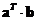or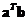. Vector inner product is also called vector scalar product because the result of the vector multiplication is a scalar. Vector inner product is closely related to matrix multiplication . It can only be performed for two vectors of the same size.

Geometrically, vector inner product measures the cosine angle between the two input vectors.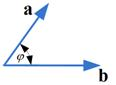Algebraically, the vector inner product is a multiplication of a row vector by a column vectorto obtain a real value scalar provided by formula below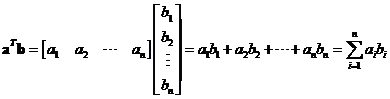Some literature also use symbol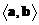to indicate vector inner product because the in the computation, we only perform sum product of the corresponding element and the transpose operator does not really matter. In this linear algebra tutorial, I use notationfor vector inner product to distinct the inner product from the outer product .

Example
Suppose we have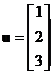and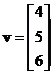, the vector inner product is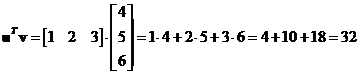Try the interactive program of Vector Inner Product below. To use the program, simply click the "Vector Inner Product" button. "Random example" button will give you unlimited examples of the vectors in the right format. You can type your own input vectors.

vector x vector y

## Properties

Some important properties of vector inner product are

• Vector inner product is a commutative operation. If you reverse the order you will get the same result but you should notice the transpose operator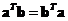• Vector inner product is a distributive operation. You can distribute (and group) the first vector with respect to addition or subtraction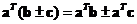• Vector inner product is an associative operation with respect to scalar multiple of vector . You can exchange the order of computation (operation inside parentheses are to be computed first) does not change the result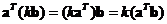.
• Vector dot product to itself always produces positive number except when it is a zero vector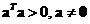. It produces zero if and only if the vector input is a zero vector.
• Square of Euclidean norm of a vector is equal to the inner product to itself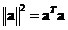• Cosine angle between two vectors is equal to their dot product divided by the product of their norms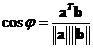• Two vectors are perpendicular (orthogonal) to each other if and only if their inner product is zero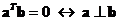.
• Dot product of the same standard unit vector is one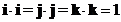• Dot product of the perpendicular standard unit vector is zero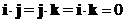• Relationship of norm of cross product and dot product is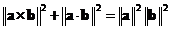.

See also: Cross product , Outer Product

< Next | Previous | Index >